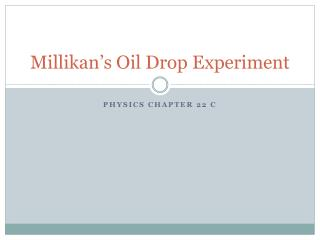DownloadDownload PresentationMillikan’s Oil Drop Experiment

# Millikan’s Oil Drop Experiment

Télécharger la présentation## Millikan’s Oil Drop Experiment

- - - - - - - - - - - - - - - - - - - - - - - - - - - E N D - - - - - - - - - - - - - - - - - - - - - - - - - - -
##### Presentation Transcript

1. Millikan’s Oil Drop Experiment Physics Chapter 22 C

2. Atoms • By the early 1900s, we knew: • Atoms had electrons in them (J. J. Thomson) • Atoms had positive charges in them (protons) • Structure???

3. Millikan’s Experiment • Milikan Oil Drop • Millikan

4. Millikan’s Experiment • Experiment balanced gravity and charges • Eq = mg • If E is known due to voltage (V = Ed) • And weight is determined • We can find q • Q was found to be a multiple of 1.6 x 10-19 C

5. Example p375 • An oil drop weighs 1.9 x 10-14 N. It is suspended in an electric field of intensity 4.0 x 104 N/C. What is the charge on the oil drop? • What we know: • W = 1.9 x 10-14 N E = 4.0 x 104 N/C • Equation: • Q = W/E • Substitute: • Q = (1.9 x 10-14 N)/(4.0 x 104 N/C) • Math: • Q= 4.75 x 10-19 C

6. Example p 375 continued • If the drop is attracted toward the positive plate, how many excess electrons does it have? • What we know: • Q = 4.75 x 10-19 C e- charge: 1.6 x 10-19 C • Equation: • # e- = q/charge of e- • Substitute: • #e- = (4.75 x 10-19 C)/(1.6 x 10-19 C) • Math: • #e- = 2.9688 = 3